# How to test the power level of crystal in MAX9485-How to Te

Abstract: MAX9485 is a versaTIle, programmable, audio clock generator. It consists of a VCXO, fracTIonal-N PLL, and IÂ²C interface. To use the MAX9485 appropriately, it is important that the power level of the VCXO's crystal does not exceed its maximum raTIng. This applicaTIon note describes a simple method for measuring the power level of a crystal oscillator, and reports the test results on the MAX9485.IntroductionThe MAX9485 [1, 2] is a cost-effective, programmable, multiple-output audio clock generator for MPEG-2 or AC-3 audio systems such as DVD players, a DVD drive for multimedia PC's, HDTV's, home entertainment centers, and set-top boxes. The MAX9485 has a fractional-N programmable PLL with two output drivers, a VCXO with Â± 200 PPM pulling range, and an IÂ²C interface. Its system functional diagram is shown in Figure 1.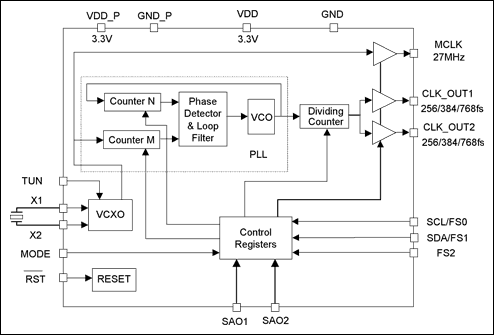Figure 1. Functional diagram of the MAX9485 clock generator.

When using the MAX9485, the crystal's power level should be less than the maximum rating given by the crystal's manufacturer. If the crystal's driving level is higher than its maximum rating, the crystal's equivalent serial resistance (ESR) and frequency accuracy may degrade. If the crystal's power were 10x over its maximum rating, it could cause irreversible damage to the crystal.

In this application note, we first review the formula for calculating a crystal's power level. We then introduce a simple test setup for measuring the crystal's power level, and finally present the test result from the MAX9485. Calculation of a Crystal's Power LevelFigure 2 illustrates a circuit that functions equivalent to a crystal .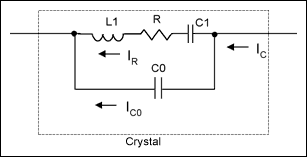Figure 2. Circuit diagram of a crystal Equivalent.

The ESR R, shown in Figure 2, of a crystal causes the crystal to consume power. If the RMS (root of mean square) current going through R is IR, then we can obtain the crystal's power level by;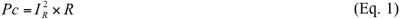However, in practice, IR is not measurable. When a crystal is resonating, the impedance of the LC branch is much smaller than that of the C0 branch. Therefore, we can use IC to approximate IR. Thus, we get a practical formula for the crystal's power-level calculation: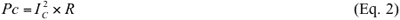The current going through the crystal is AC and its waveform is often sinusoidal or saw-tooth. The following relations determine the RMS values â€‹â€‹of sine-wave and saw-tooth wave currents from their peak-to-peak amplitudes.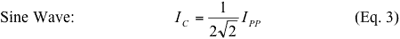where IPP is the peak-to-peak value of the current. Crystal Power-Level Test Setup For the MAX9485, we recommend that you use the 27MHz crystal (DSX530GA)  from KDS America. This crystal has a rated maximum power level of 300ÂµW.

Measuring the crystal's current is not straightforward. In the test setup shown in Figure 3, we use a small 10Î© resistor to sense the current. We know that using the 10Î© resistor is valid, because changing the resistor to 20Î© barely changed the sensed current.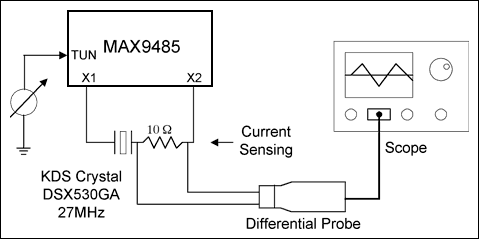Figure 3. Setup for testing a crystal's power level. Measurement Data and Power-Level CalculationUsing the test setup in Figure 3, the highest crystal power level occurs at 27MHz, which is around the center of the VCXO tuning range. The following plots (Figures 4, 5, and 6) are the measured voltage waveforms across the current-sensing resistor at different frequencies. To reduce the measurement noise, the scope's 250MHz lowpass filter is turned on.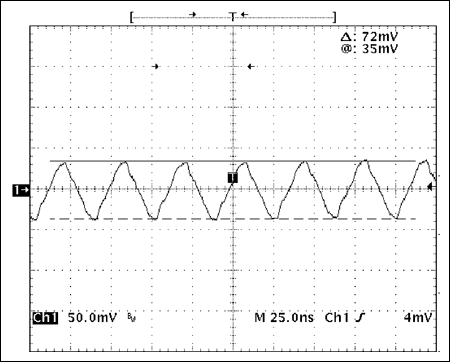Figure 4. Voltage waveform across the 10Î© resistor at 26.99500MHz (-185PPM).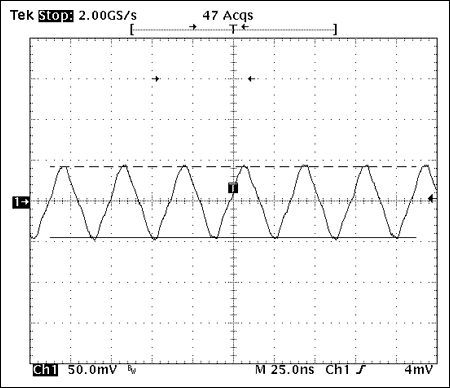Figure 5. Voltage waveform across the 10Î© resistor at 27.00000MHz (0PPM).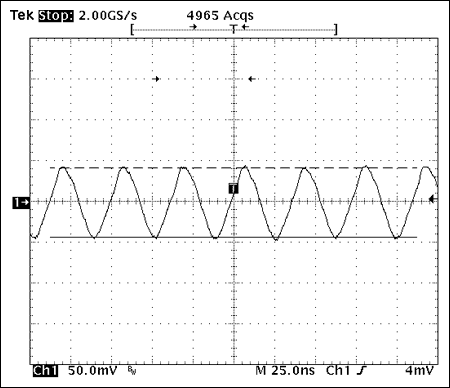Figure 6. Voltage waveform across the 10Î© resistor at 27.00400MHz (148PPM).

In calculating the crystal's power level, we consider two scenarios: (1) the worst case (that is, the maximum R value), and (2) the typical case.

The R value shown in the DSX530GA data sheet is 50Î©. This is, however, the absolute maximum for the 12MHz to 54MHz frequency range. Typically, the higher the crystal frequency, the less the R value. We consulted with KDS America and were informed that for a 27MHz DSX530GA crystal, the maximum R value (case # 1) is less than 40Î©, and the typical value (case # 2) is 20Î©. As shown in the plots above, the crystal currents have saw-tooth waveforms. We thus use Equation 4 to calculate the crystal RMS current from its peak-to-peak value. Therefore, the crystal's calculated power levels are shown in Table 1.

Table 1. Measured Results and Crystal Power Levels
 Frequency MHz / PPM Peak-to-Peak Voltage on 10Î© (mV) Peak-to-Peak Current (mA) RMS (1) Current (IC) Max Power (mÎ©) ICÂ² x R R = 40Î© Typ Power (mÎ©) ICÂ² x R R = 20Î© 26.995 / -185 72 7.2 3.6 /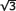172.8 86.4 27.000 0 88 8.8 4.4 /258.13 129 27.004 / 148 86 8.6 4.3 /246.5 123.2

Note: (1) The RMS value of a saw-tooth waveform is 1/2of the peak-to-peak value. ConclusionsWe can make some observations from Table 1. In the worst case, the crystal power level of the MAX9485 is about 40ÂµW less than the maximum power level (300ÂµW) of DSX530GA. In the typical case, with the realistic value of R at 27MHz, the power level is well under the maximum power level (300ÂµW). For the VCXO in the MAX9485, the highest crystal current occurs at the middle of the tuning range and the power variation is relatively small within the tuning range. Reference
 MAX9485 data sheet
 MAX9485 application note 2097, "Using a VCXO (Voltage-Controlled Crystal Oscillator) as a Clock (CLK) Generator."
 See the application note 2127, "Modeling of Quartz Crystal."
 DSX530GA data sheet

C7/C9 Light Bulb LED Filament COB technology provides amazing illumination. The LED filament bulb tough polystyrene construction provides super bright and shatterproof.Liven up your light display for the holidays with these luxury bulbs! Install these Bulbs into screw base fixtures directly. Unlike fluorescent bulbs, these bulbs are free of UV and mercury.

C7/C9 Light Bulb

Pink Led Bulb,Colored Light Bulb,E12 Replacement Bulb,Purple Light Bulb

DONGGUAN JIANXING LIGHTING ELECTRIC APPLIANCES CO., LTD , https://www.rslightstring.com